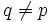# Analogue of Thompson transitivity theorem fails for abelian subgroups of rank two

It is possible to choose a finite group$G$ and a prime number$p$ such that$G$ is a group in which every p-local subgroup is p-constrained, such that:
There exists a subgroup$A$ of$G$ that is maximal among abelian normal subgroups in some$p$-Sylow subgroup of$G$, such that$A$ has rank two, and there is a prime$q \ne p$ such that$C_G(A)$ is not transitive on the collection of maximal$A$-invariant$q$-subgroups of$G$.